#### Scale: 10-13 meters = 100 fm = 100 femtometers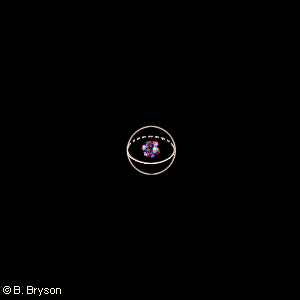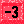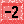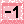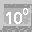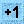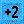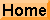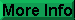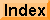## The Nucleus

The tiny nucleus of stable carbon contains 12 nucleons (protons and neutrons). How much larger would the nucleus be for a uranium atom, for example 238U? A simple calculation shows that even the largest nuclei would fit within a sphere with a diameter not much larger than the diagonal of one of the small center squares. Recall that the volume of a sphere is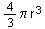. If we let r be the radius of the carbon nucleus, then the white sphere in the picture has radius 3r. So the volume of the sphere is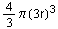, or 27(), 27 times the volume of the carbon nucleus. Since there are 12 nucleons in the carbon nucleus, we could squeeze as many as 27 x 12 = 324 nucleons inside the white sphere – easily more than enough for 238U.